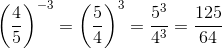# GED Math : Exponents

## Example Questions

← Previous 1 3 4 5 6

### Example Question #1 : Exponents

Solve: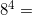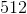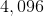Explanation: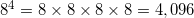### Example Question #2 : Exponents

Write the following expression in expanded form: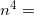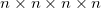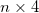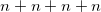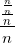Explanation:

An exponent indicates the amount of times a number (or variable) should be multiplied by itself. For example,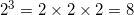.

In this instance,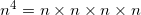.

### Example Question #471 : Ged Math

Simplify: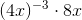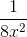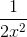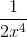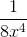Explanation: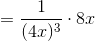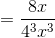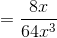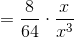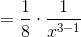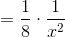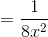### Example Question #472 : Ged Math

Express 45,000,000 in scientific notation.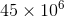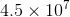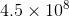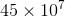Explanation:

Write the number with a decimal point and without commas: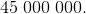Counting the number of places, move the decimal point to the left until it is after the first nonzero digit: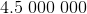The resulting  number is 4.5; the number of places the decimal point moved to the left was 7. In scientific notation, this number is.

### Example Question #473 : Ged Math

Express 0.000000038 in scientific notation.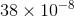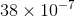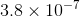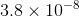Explanation:

Write the number: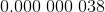Counting the number of places, move the decimal point to the right until it is after the first nonzero digit: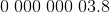The resulting  number is 3.8; the number of places the decimal point moved to the right was 8. In scientific notation, this number is.

### Example Question #474 : Ged Math

Which of the following is not equal to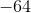?

Do not use a calculator.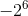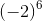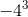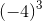Explanation:

A positive number raised to any real power is positive.andare the opposites of powers of positive numbers; they are therefore the opposites of positive numbers, making both negative.

A negative number raised to an odd power, such as, is negative.

A negative number raised to an even power, such as, is positive.is the only positive number among the choices and can be deduced as the correct choice.

### Example Question #475 : Ged Math

Without using a calculator, evaluate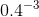.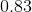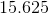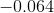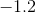Explanation:

For any nonzeroand for any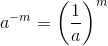. Equivalently, any number raised to a negative power is equal to the reciprocal of that number raised to the power of the absolute value of the exponent.

The reciprocal of a number is the quotient of 1 and the number, so the reciprocal of 0.4 is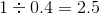.

Therefore,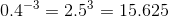, which is the correct response.

### Example Question #476 : Ged Math

Evaluate without using a calculator: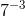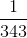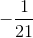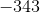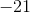Explanation: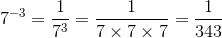### Example Question #477 : Ged Math

Simplify the expression: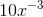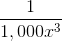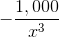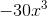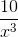Explanation:

A number or variable can be raised to a negative power by taking the reciprocal of the same number raised to the power of the absolute value of the exponent.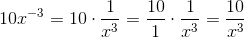### Example Question #478 : Ged Math

Without using a calculator, evaluate: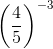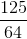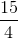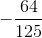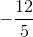For any nonzeroand for any. Equivalently, any number raised to a negative power is equal to the reciprocal of that number raised to the power of the absolute value of the exponent. Apply this power, along with the power of a quotient property: